# Problem 51087. Solve an ODE: equation for a 2D laminar jet

Problem
In the solution for a two-dimensional laminar jet, the following nonlinear ordinary differential equation arises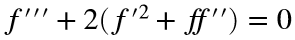where primes denote differentiation with respect to the independent variable η. The problem has the conditions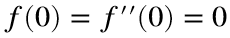and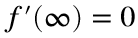and the constraintwhere a is a constant.
Write a function to solve this problem—that is, return values of f at specified values of η. The test suite allows MATLAB’s functions for numerical solution of ODEs, but the equation has a relatively simple analytical solution. If you would like a hint for the analytical solution, execute this command:
char('Zxj%ymj%uwtizhy%wzqj%tk%inkkjwjsynfynts%y|t%ynrjx%fsi%nsyjlwfyj%ymwjj%ynrjx3'-5)
Background
The physical problem involves a jet in the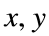plane emanating from a source at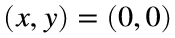. It can be solved by expressing the velocities u and v in terms of a streamfunction and employing a similarity solution that combines the spatial coordinates into a single variable η. In the problem above,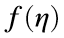is proportional to the streamfunction. The conditions at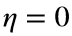define the line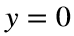as a line of symmetry; the transverse velocity v and the shear stress are zero there. The conditionstates that the streamwise velocity u is zero far from the source. The integral constraint states that the momentum flux of the jet is constant. The value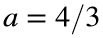corresponds to the physical problem of the jet. Other values are included for variety.

### Solution Stats

66.67% Correct | 33.33% Incorrect
Last Solution submitted on Apr 28, 2022

### Community Treasure Hunt

Find the treasures in MATLAB Central and discover how the community can help you!

Start Hunting!